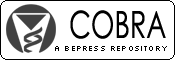## COBRA Preprint Series

#### Abstract

The existence of maximum likelihood estimates for the binary response logistic regression model depends on the configuration of the data points in your data set. There are three mutually exclusive and exhaustive categories for the configuration of data points in a data set: Complete Separation, Quasi-Complete Separation, and Overlap. For this paper, a binary response logistic regression model is considered. A 2 x 2 tabular presentation of the data set to be modeled is provided for each of the three categories mentioned above. In addition, the paper will present an example of a data set whose data points have a linear dependency. Both unconditional maximum likelihood estimation (asymptotic inference) and exact conditional estimation (exact inference) will be considered and contrasted in terms of results. The statistical software package SAS will be used for the binary response logistic regression modeling.

Biostatistics

COinS